Test: Series-Parallel Interconnection of Two Port Network

# Test: Series-Parallel Interconnection of Two Port Network

Test Description

## 15 Questions MCQ Test Network Theory (Electric Circuits) | Test: Series-Parallel Interconnection of Two Port Network

Test: Series-Parallel Interconnection of Two Port Network for Electrical Engineering (EE) 2023 is part of Network Theory (Electric Circuits) preparation. The Test: Series-Parallel Interconnection of Two Port Network questions and answers have been prepared according to the Electrical Engineering (EE) exam syllabus.The Test: Series-Parallel Interconnection of Two Port Network MCQs are made for Electrical Engineering (EE) 2023 Exam. Find important definitions, questions, notes, meanings, examples, exercises, MCQs and online tests for Test: Series-Parallel Interconnection of Two Port Network below.
Solutions of Test: Series-Parallel Interconnection of Two Port Network questions in English are available as part of our Network Theory (Electric Circuits) for Electrical Engineering (EE) & Test: Series-Parallel Interconnection of Two Port Network solutions in Hindi for Network Theory (Electric Circuits) course. Download more important topics, notes, lectures and mock test series for Electrical Engineering (EE) Exam by signing up for free. Attempt Test: Series-Parallel Interconnection of Two Port Network | 15 questions in 45 minutes | Mock test for Electrical Engineering (EE) preparation | Free important questions MCQ to study Network Theory (Electric Circuits) for Electrical Engineering (EE) Exam | Download free PDF with solutions
 1 Crore+ students have signed up on EduRev. Have you?
Test: Series-Parallel Interconnection of Two Port Network - Question 1

### For the circuit given below, the value of z21 parameter is ____________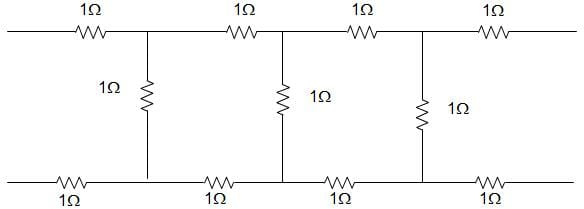Detailed Solution for Test: Series-Parallel Interconnection of Two Port Network - Question 1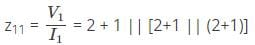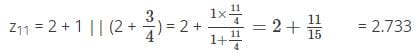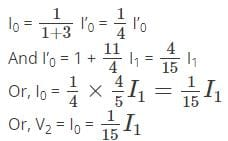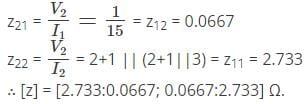Test: Series-Parallel Interconnection of Two Port Network - Question 2

### Consider a cube having resistance R on each of its sides. For this non-planar graph, the number of independent loop equations are _______________

Detailed Solution for Test: Series-Parallel Interconnection of Two Port Network - Question 2

We know that the number of equations is given by,
L = B – N + 1
Where, B = Number of Branches, N = Number of Nodes
Here, B = 12 and N = 8.
So, L = 12 – 8 + 1 = 5.

Test: Series-Parallel Interconnection of Two Port Network - Question 3

### Consider a series RL circuit in which current 12 A is flowing through R and current 16 A is flowing through L. The current supplied by the sinusoidal current source I is ____________

Detailed Solution for Test: Series-Parallel Interconnection of Two Port Network - Question 3

Current I (t) is given by,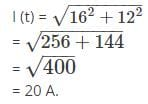Test: Series-Parallel Interconnection of Two Port Network - Question 4

Two coils X and Y have self-inductances of 5 mH and 10 mH and mutual inductance of 3 mH. If the current in coils X change at a steady rate of 100 A/s, the emf induced in coil Y is ____________

Detailed Solution for Test: Series-Parallel Interconnection of Two Port Network - Question 4

The emf is given by,Hence, the emf induced in coil Y is given by 0.3 V.

Test: Series-Parallel Interconnection of Two Port Network - Question 5

The Thevenin’s equivalent of a network is a 10 V source in series with 2 Ω resistances. If a 3 Ω resistance is connected across the Thevenin’s equivalent is _____________

Detailed Solution for Test: Series-Parallel Interconnection of Two Port Network - Question 5

The Thevenin equivalent voltage is given by,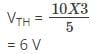And the Thevenin equivalent Resistance is given by,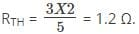Test: Series-Parallel Interconnection of Two Port Network - Question 6

A parallel RLC circuit with R1 = 20, L1 = 1/100 and C1 = 1/200 is scaled giving R2 = 104, L2 = 10-4 and C2, the value of C2 is ___________

Detailed Solution for Test: Series-Parallel Interconnection of Two Port Network - Question 6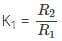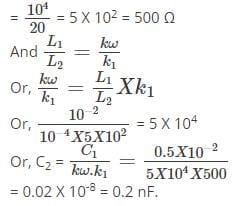Test: Series-Parallel Interconnection of Two Port Network - Question 7

Consider an RL series circuit having resistance R = 3 Ω, inductance L = 3 H and is excited by 6V. The current after a long time after closing of switch is ____________

Detailed Solution for Test: Series-Parallel Interconnection of Two Port Network - Question 7

At t = ∞ the circuit has effectively two 6Ω resistances in parallelGiven voltage = 6 V
So, current = 2 A.

Test: Series-Parallel Interconnection of Two Port Network - Question 8

For the circuit given below, the value of z11 parameter is ____________

Detailed Solution for Test: Series-Parallel Interconnection of Two Port Network - Question 8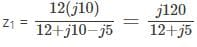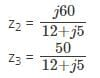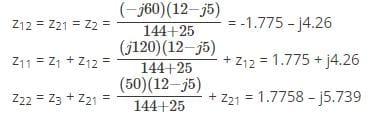∴ [z] = [1.775 + j4.26; -1.775 – j4.26; -1.775 – j4.26; 1.775 – j5.739] Ω.

Test: Series-Parallel Interconnection of Two Port Network - Question 9

Given two voltages, 50 ∠0 V and 75 ∠- 60° V. The sum of these voltages is ___________

Detailed Solution for Test: Series-Parallel Interconnection of Two Port Network - Question 9

The voltages can be written in the form,
50 + j 0.75∠-60°
= 37.5 – j 64.95
So, sum = (50 + 37.5) – j 64.95
= 87.5 – j 64.95 = 109∠-36.6°.

Test: Series-Parallel Interconnection of Two Port Network - Question 10

Consider a circuit having resistances 16 Ω and 30 Ωis excited by a voltage V. A variable resistance R is connected across the 16 Ω resistance. The power dissipated in 30 Ω resistance will be maximum when value of R is __________

Detailed Solution for Test: Series-Parallel Interconnection of Two Port Network - Question 10

We know that,
When R = 0, circuit current = V/30A
And Power dissipated = V2/30 Watts.
This is the maximum possible value which occurs for R = 0 Ω.

Test: Series-Parallel Interconnection of Two Port Network - Question 11

A 50 Hz current has an amplitude of 25 A. The rate of change of current at t = 0.005 after i = 0 and is increasing is ____________

Detailed Solution for Test: Series-Parallel Interconnection of Two Port Network - Question 11

The current i (t) is given by,
i = 25 sin 314.16 t and di/dt = 250 X 314.16 cos⁡ωt
Now, at t = 0.005, I = 25 X 314.16 cos (314.16 X 0.005)
= 0.

Test: Series-Parallel Interconnection of Two Port Network - Question 12

A magnetic circuit has an iron length of 100 cm and air gap length 10 cm. If μr = 200 then which of the following is true?

Detailed Solution for Test: Series-Parallel Interconnection of Two Port Network - Question 12

We know that, MMF for air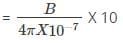Where B is the magnetic field intensity.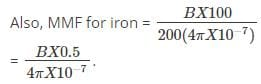Test: Series-Parallel Interconnection of Two Port Network - Question 13

Barletts Bisection Theorem is applicable to ___________

Detailed Solution for Test: Series-Parallel Interconnection of Two Port Network - Question 13

A symmetrical network can be split into two halves. So the z parameters of the network are symmetrical as well as reciprocal of each other. Hence Barletts Bisection Theorem is applicable to Symmetrical networks.

Test: Series-Parallel Interconnection of Two Port Network - Question 14

The energy stored in a coil is 108 J. The power dissipated instantaneously across the blades of switch after it is opened in 10 ms is ____________

Detailed Solution for Test: Series-Parallel Interconnection of Two Port Network - Question 14

Power dissipated instantaneously across the blades of the switch is given by,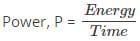Given that, Energy = 108 J and time = 10 X 10-3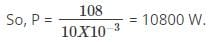Test: Series-Parallel Interconnection of Two Port Network - Question 15

If the diameter of a wire is doubled, the current carrying capacity of the wire is ___________

Detailed Solution for Test: Series-Parallel Interconnection of Two Port Network - Question 15

Since diameter is doubled, area of cross-section becomes four times. Current carrying capacity is proportional to area of cross-section.

## Network Theory (Electric Circuits)

23 videos|63 docs|60 tests
 Use Code STAYHOME200 and get INR 200 additional OFF Use Coupon Code
Information about Test: Series-Parallel Interconnection of Two Port Network Page
In this test you can find the Exam questions for Test: Series-Parallel Interconnection of Two Port Network solved & explained in the simplest way possible. Besides giving Questions and answers for Test: Series-Parallel Interconnection of Two Port Network, EduRev gives you an ample number of Online tests for practice

## Network Theory (Electric Circuits)

23 videos|63 docs|60 tests